# Angol nyelvű matematika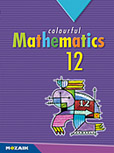## Colourful Mathematics 12.

12. évfolyam, 2. kiadás (2019. 04. 11.)

kód: MS-6312
ára: 4 980 Ft
méret: B5, 288 oldal
Kosárba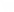TartalomjegyzékMintaoldalakSegédanyag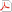Emelt színtű matematika érettségi témakörök (2020)Kapcsolódó kiadványok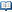MS-6309 - Colourful Mathematics 9.MS-6310 - Colourful Mathematics 10.MS-6311 - Colourful Mathematics 11.MS-2312 - Sokszínű matematika 12.interaktív táblára CLASSROOM Digitális változat elérhetőweb-tankönyv HOME Digitális változat elérhető
 A kiadvány az MS-2312 Sokszínű matematika 12. c. kötet angol nyelvű változata. This book is the English version of the Hungarian market leader textbook titled Sokszínű matematika 12.
 Digitális változat egyedi kóddal*A kiadvány hátsó borítójának belső oldalán található egyedi kóddal a kiadvány digitálisan is elérhető. Az aktivált kódokkal DÍJMENTES hozzáférést biztosítunk a kiadvány mozaWeb Home változatához az aktiválástól számított minimum egy éves időtartamra. A kódok csak egyszer aktiválhatók.
Mintaoldalak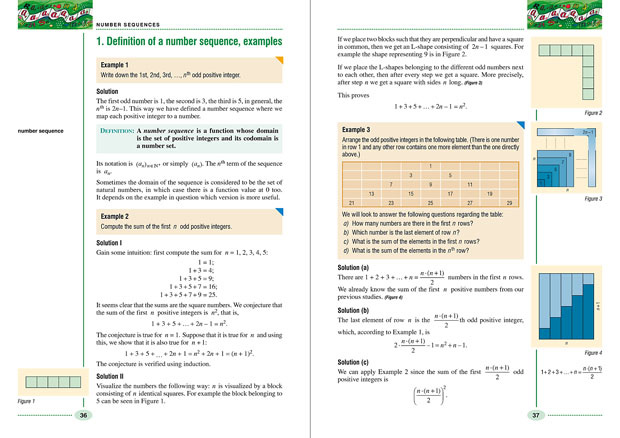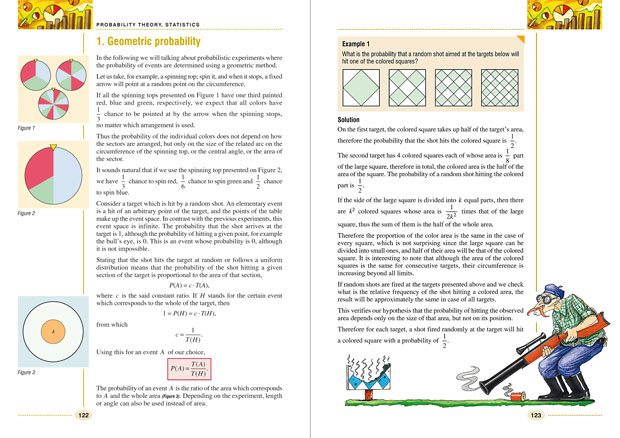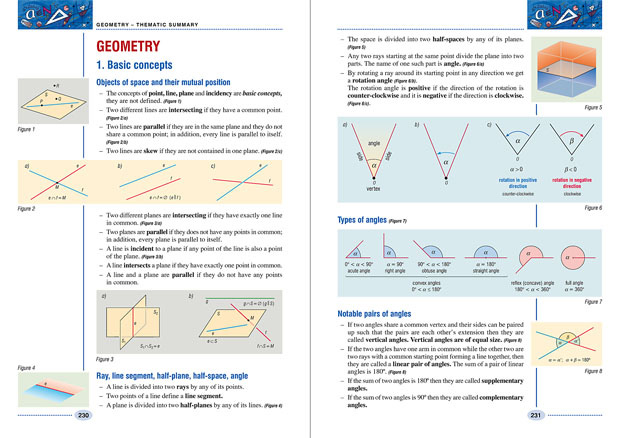Tartalomjegyzék
 Logic and methods of proof 9 1. Logic problems and statements 10 2. Logical operators: negation, conjunction, disjunction 15 3. Logical operators: implication and equivalence 23 4. Induction (higher level courseware) 28 Number sequences 35 1. Definition of a number sequence, examples 36 2. Recursive sequences – examples 41 3. Arithmetic progressions 48 4. Geometric progressions 54 5. Interest calculation, computation of instalments 61 Three-dimensional geometry 65 1. Geometric objects in space 66 2. Division of the plane and the space (extra-curricular topic) 72 3. Classification of solids, Platonic solids 76 4. The concept of area, area of polygons 82 5. The area of the disc and its parts 87 6. Concept of volume, the volume of the prism and the cylinder 92 7. Volume of the pyramid and the cone 98 8. Conical and pyramidal frustum 103 9. The volume and surface of the sphere 108 10. Inscribed solids (extra-curricular topic) 112 11. Applications of three-dimensional geometry 118 Probability theory, statistics 121 1. Geometric probability 122 2. Expected value (higher level courseware) 128 3. Statistics 134 Thematic summary 145 Methods of reasoning 146 1. Sets, statements, events 146 2. Combinatorics, probability 152 Algebra and number theory 161 1. Numbers and operations 161 2. Number theory, divisibility 164 3. Powers, root, logarithm 167 4. Operations with rational expressions 178 5. Equations, inequalities 183 6. Simultaneous equations (systems of equations) 213 Functions 218 1. Definition and graph of a function, simple properties 218 2. Operations on functions (extra-curricular topic) 221 3. Properties of functions 224 Geometry 230 1. Basic concepts 230 2. Geometric transformations 238 Congruent transformations 238 Similarity transformation 243 3. Vectors. Trigonometric functions 248 4. Properties of notable planar objects 257 5. Coordinate geometry 270 Problem sets for intermediate level maturity exam 276 Problem set 1 277 Problem set 2 279 Problem set 3 281 Problem set 4 283 Problem set 5 286